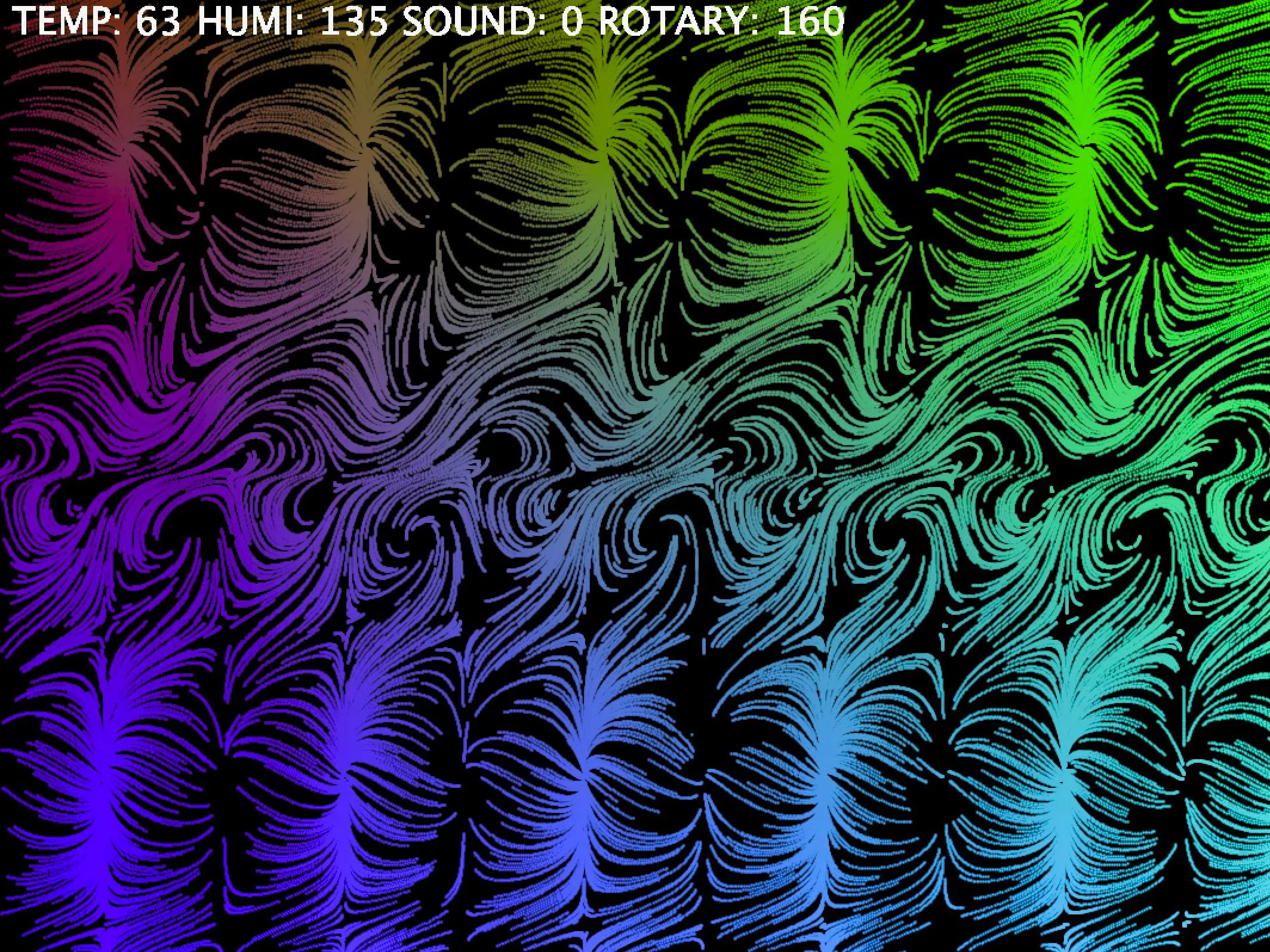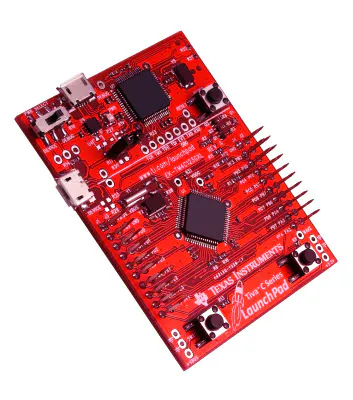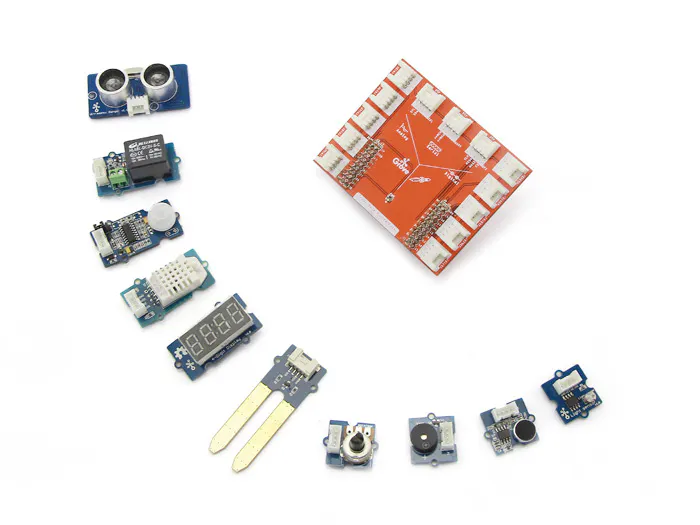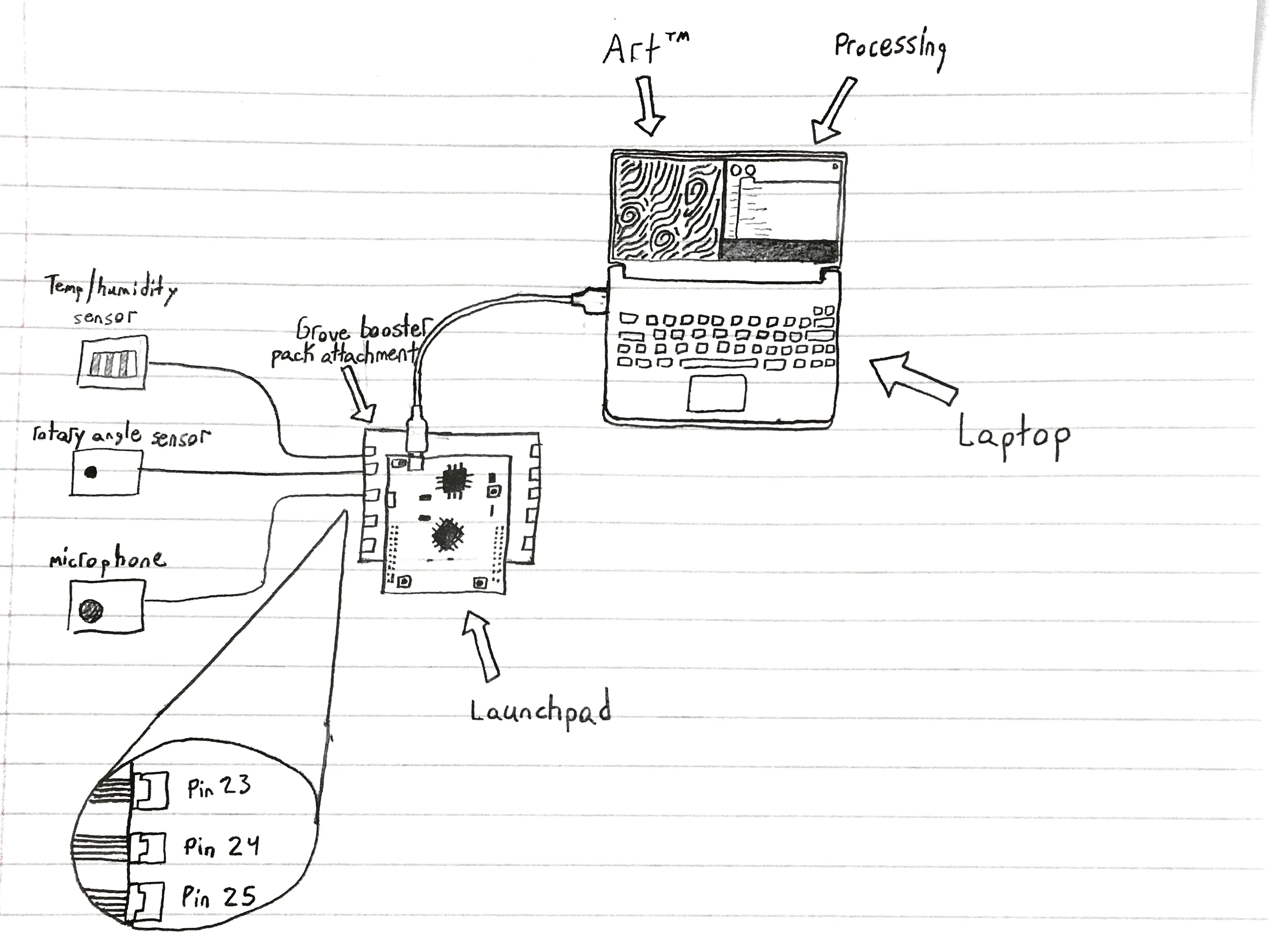Published

# Wacky Weirdos: Creating Art with Data

Our project turns environmental data (temperature, humidity, sound) into beautiful works of mathematical art.

IntermediateShowcase (no instructions)8,467## Things used in this project

### Hardware componentsTexas Instruments EK-TM4C123GXL TM4C Tiva LaunchPad
×1Seeed Grove Starter Kit for LaunchPad
×1

### Software apps and online servicesThe Processing Foundation ProcessingTexas Instruments Energia
 Apple macOS

## Schematics

### Simple Schematic## Code

### Processing Code

Processing
This is the code that will render the art on your computer. Make sure to download and install processing. It may require you to also install java if you don't already have it.

NOTE: Some of this code is based on sample code available as examples in the program itself. https://processing.org/tutorials/pvector/
```// IMPORTS NECESSARY FOR SERIAL COMMUNICATION
import org.quark.jasmine.*;
import processing.serial.*;

float e = 2.718281828459045;
float counter = 0;

// Number inside of array determines how many particles rendered on the screen
// Lower / Raise this number depending on your computer. 40,000 is about the max on my computer before
// my computer starts to choke. A gaming laptop can probably handle quite a bit more.
Mover[] movers = new Mover;
Serial myPort; // The serial port object necessary for reading in values.

//Global Integers track values from each of the corresponding sensors.
// By default, they're all set to half. Each value was normalized on the board to be between 0-255, 0 being no signal, 255 meaning maximum signal.
int temp = 127;
int humi = 127;
int sound = 127;
int toggle = 127;

//Runs once at startup, just like Energia
void setup () {
//P2D allows faster rendering on some computers. Remove the line inside the parenthesis if it doesn't work / isn't compatible with your computer
fullScreen(P2D);
//Prints out list of serial ports. THIS WILL VARY DEPENDING ON COMPUTER.
//Not sure if this will work on windows, but play around with the correct serial port until serial communication works
println(Serial.list());
//Set Serial.list[NUMBER] according to the corresct serial port attached
myPort = new Serial(this, Serial.list(), 9600);
//Looks for new line character
myPort.bufferUntil('\n');
//Sets background color to black.
background(0);
//Initialized mover objects (particles) according to number allocated at top of code
for (int i = 0; i < movers.length; i++) {
movers[i] = new Mover();
}

}

//This giant code block loops pretty much as quickly as your computer can handle it.
void draw(){

//This determines if "particles" or "lines" are drawn on the screen. Uses the value input from the mic.
// I found that using 28 was a good balance for the mic we used. Essentially, if the sound reading is
//greater than 28, it will redraw the background every frame. If below, it never removes the last frame creating a path
if (sound > 28){
background(0);
//Stroke weight determines radius of points drawn
strokeWeight(2);
} else {
strokeWeight(1);
}

//This code should hopefully work on your computer. Creates new threads to update the points, that way the processor putting
// drawing the image isn't bogged down waiting for new calculations

//Loops through each particle / mover to display them on the screen.
for (int i = 0; i < movers.length; i++) {
movers[i].display();
}

//Draws the information about the sensor readings in the top left corner of the screen.
textSize(32);
text("TEMP: " + temp + " HUMI: " + humi + " SOUND: " + sound + " ROTARY: " + toggle, 10, 30);
fill(256,256,256);

//Checks if spacebar has been presses
if (keyPressed){
if (key == ' ')
//Checks the Serial Buffer to see if anything is avaliable to read
if ( myPort.available() > 0) {
//Read in the value from the serial port as a string. This value will be 8 characters long, every two representing a number
// between 0 and 255 in HEX
//Keep this delay here. Dealing with serial ports is pretty annoying and rushing it will cause things to crash :(
delay(150);
//Clears the Serial Buffer.
myPort.clear();
if (inString.length() > 0) {
//
// First Pair of Charactes = Hex Value for temperature reading
// Second Pair of Characters = Hex Value for humidity reading
// Third Pair of Characters = Hex Value recorded from mic
// Fourth Pair = Hex Value for Rotary Angle Sensor
inString = trim(inString);
//Decodes each of the Hex values
temp = Integer.decode("0x" + inString.substring(0,2));
humi = Integer.decode("0x" + inString.substring(2,4));
sound = Integer.decode("0x" + inString.substring(4,6));
toggle = Integer.decode("0x" + inString.substring(6,8));

// Wipes out the background to draw the new vector field
background(0);
//Resets the position of all the particles Allows him to interact with vector field.
for (int i = 0; i < movers.length; i++) {
movers[i].reset();
}
}
}
}
}
}

//Starts Thread 1 for calculating the new position for 1/4th of the points.
public void update_points_1(){
for (int i = 0; i < movers.length/4; i++) {
movers[i].update(humi,temp,sound,toggle);
movers[i].checkEdges();
}
}

//Starts Thread 2 for calculating the new position for 1/4th of the points.
public void update_points_2(){
for (int i = movers.length/4; i < movers.length/2; i++) {
movers[i].update(humi,temp,sound,toggle);
movers[i].checkEdges();
}
}

//Starts Thread 3 for calculating the new position for 1/4th of the points.
public void update_points_3(){
for (int i = movers.length/2; i < 3*(movers.length)/4; i++) {
movers[i].update(humi,temp,sound,toggle);
movers[i].checkEdges();
}
}

//Starts Thread 4 for calculating the new position for 1/4th of the points.
public void update_points_4(){
for (int i = 3*(movers.length)/4; i < movers.length; i++) {
movers[i].update(humi,temp,sound,toggle);
movers[i].checkEdges();
}
}

class Mover{

//Vector Objects for tracking location and velocity.
PVector location;
PVector velocity;
float fx; // Derivative in i-hat direction
float fy; // Derivative in j-hat direction

//Saves values from sensors
float mTemp;
float mSound;
float mHumi;
float mToggle;

//Creates mover objects. Set random location on the screen. Sets velocity to zero
Mover() {
location = new PVector(random(width),random(height));
velocity = new PVector(0,0);
}

//Resets the location of the mover and sets the velocity to zero
void reset(){
location = new PVector(random(width),random(height));
velocity = new PVector(0,0);
}

//Function called by the threads to update the particles / movers
void update(int humi, int temp, int sound, int toggle) {
//Updates values in object. I copy the values because I found some weird bugs when using threading.
mTemp = (float) temp;
mSound = (float) sound;
mToggle = (float) toggle;
mHumi = (float) humi;

//I map the vector's location to a value between -20 and 20. This makes typing in vector field equations easier.
float x = (map(location.x, 0, width, -200, 200))/10.0;
float y = (map(location.y, 0, height, -100, 100))/10.0;

//Monster sized piece wise function. Mathematically described in the project writeup. Basically uses the different values from
// the sensors to decide the properties of the vector field.
if (mToggle >= 0) {
fx = sin(x + y);
fy = cos(x + y);
}
if (mToggle > 100){
fx = sin(x - y);
fy = sin(x + y);
}
if (mToggle > 150){
fx = sigmoid(y) + cos(x)*log(abs(y));
fy = sigmoid(x/10);
}
if (mToggle > 170){
fx = log(abs(y));
fy = log(abs(x));
}
if (mToggle > 180){
fx = log(abs(y)) - log(abs(x));
fy = log(abs(y)) + log(abs(x));
}
if (mToggle > 210){
fx = log(abs(y)) + log(abs(x));
fy = log(abs(y))*log(abs(x));
}

//Humidity modifiers
if (mHumi % 10 == 0) {
fy = cos(x + y);
}
if (mHumi % 10 == 1){
fy = sin(x + y);
}
if (mHumi % 10 == 2){
fy = -5*fy;
}

//Temperature modifiers
if (mTemp % 10 == 0) {
fx = sigmoid(sin(x))*10;
}
if (mTemp % 10 == 1){
fx = sigmoid(fy);
}
if (mTemp % 10 == 2){
fx = -5*fx;
}

// Sound modifiers
if (mSound % 7 == 0){
fx = fy;
} else if (mSound % 7 == 1){
fx = fy*-fx;
}

velocity = new PVector(fx,fy);
}

void display() {
//Sets color depending on color and location
float r = ((mTemp % 15) /5) * 256;
float g = (location.x / width) * 256;
float b = (location.y / height) * 256;
stroke(r,g,b);
//draws the point on the screen
point(location.x,location.y);
}
void checkEdges() {
//When particles go off the screen, they're respawned.
if (location.x > width) {
reset();
} else if (location.x < 0) {
reset();
}

if (location.y > height) {
reset();
}  else if (location.y < 0) {
reset();
}
}
}

//We covered this function in 182. I use it to create some pretty cool warping effects.
float sigmoid(float val){
float sig = 1;
sig = sig / (1 + pow(e,val));
return sig;
}
```

### Energia Code

C/C++
This code should be loaded on the board! It manages reading data in from the sensors as well as sending it over the serial ports.
```#include "TM1637.h"
#include "DHT.h"
#include "Ultrasonic.h"

// Different pins used to receive values
#define TEMP_HUMI_PIN 23
#define SOUND_PIN 24
#define SPIN_PIN 25
#define SEND_PIN 17

// Variables that store un-compressed sensor data
double _sound = 0;
double _toggle = 0;
double _temp = 0;
double _humi = 0;
double _dist = 0;

//Sets up Temperature Humidity Sensor
DHT dht(TEMP_HUMI_PIN, DHT22);

void setup() {
//Starts Serial communication with the computer at 9600 baud
Serial.begin(9600);
dht.begin();
}

void loop() {
//The number commented next to each value correspond the range I found I could get out of our sensors.
//The sensors aren't very high quality so often times they don't work super well.
_sound = (double) analogRead(SOUND_PIN); //0 - 4095 (MAX I CAN GET IT TO IS AROUND 2200);

//Converts each of the values to a number between 0 and 255
int t_temp = (_temp / 100 ) * 255;
int t_humi = (_humi / 100 ) * 255;
int t_sound = min((_sound / 2000) * 255,2000);
int t_dist = min((_dist / 200) * 255,200);
int t_toggle = (_toggle / 4095 ) * 255;

//After each number converted to be between 0 and 255, we bitshift them to fit into an 8 bit data type. We used an unsigned integer to store the values
unsigned int transpose = (t_temp << 24) + (t_humi << 16) + (t_sound << 8) + (t_toggle);
//Send the compressed value over the serial port in hex
Serial.println(transpose, HEX);
//Delay by 50 milliseconds.
delay(50);
}
```

## Credits

### Lucas Atayde

1 project • 0 followers

### user1051395

1 project • 0 followers

### Ranferi Avilez

1 project • 0 followers